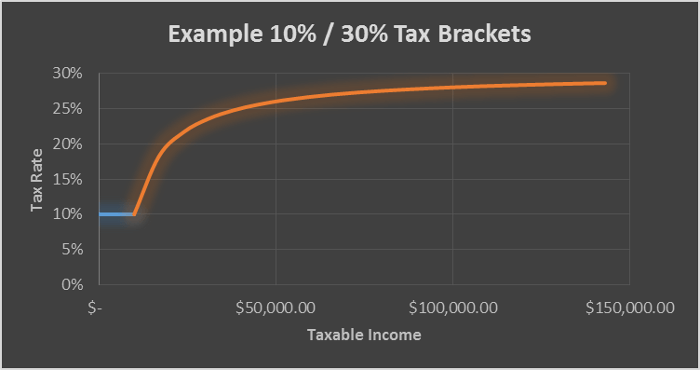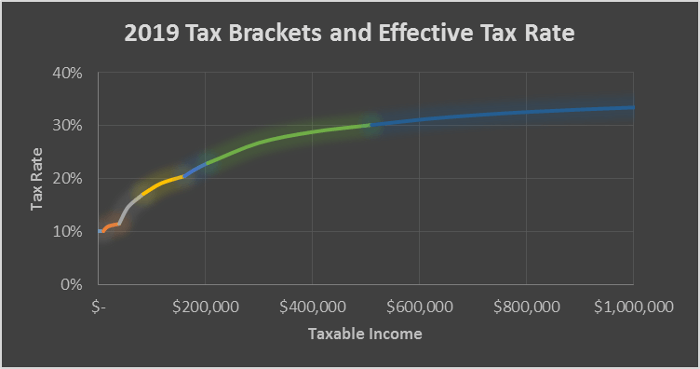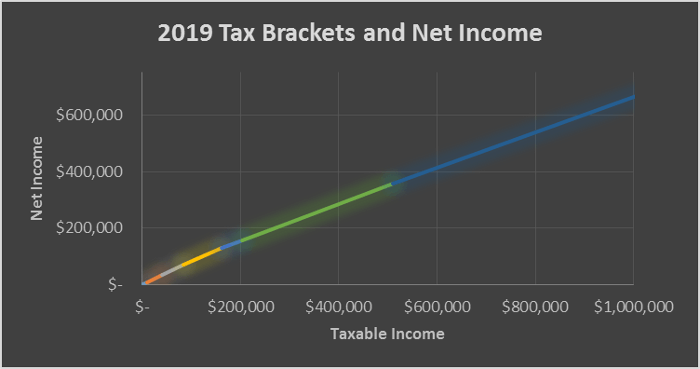Perhaps it’s old news by now, but Alexandria Ocasio-Cortez (“AOC”) recently proposed a top marginal tax of 70% on people who earn more than \$10 million. Instead of arguing the merits (or lack thereof) of this proposed tax, people instead seem to be arguing exactly what a marginal tax rate is.

A marginal tax is one that brackets income into two or more amounts, and only those amounts in that bracket are taxed at that rate (there will be an example below). A progressive marginal tax is one where the tax brackets on higher incomes are taxed more, as opposed to a regressive marginal tax where the tax brackets on lower incomes are taxed more. The US uses a progressive marginal income tax. I know of nowhere that uses a regressive model.

Confused about how marginal tax rates work in general? Check out Time In the Market’s post A Simple Explanation of How Tax Brackets Work.

The myth that crossing a tax bracket threshold means that you will make less money is strong. I first remember hearing people saying that they make less money due to taxes during my high school pizza-slinging job, and have even heard it said at my current white-collared software engineering job:

• “I actually make less now after the promotion, thanks to taxes!”
• “The extra money isn’t worth it because it’ll put you into another tax bracket, and you’ll actually bring home less money.”

However, progressive marginal tax rates do tax your entire income at a higher rate as you cross each tax bracket threshold. In fact, once you pass the first tax bracket threshold, the more you make, the higher percentage of your entire income gets taxed.

However, provided that there is never a tax bracket with a rate that is higher than 100%, it is never disadvantageous to make an additional dollar. That is, no additional income will cause the net (after-tax) income to decrease.

This is a simple mathematical fact.

## An Example Marginal Tax Rate

Let me provide an example. Assume there are but two tax brackets: a 10% tax on income earned at or below \$10,000, and a 30% tax on income earned above \$10,000. We have two individuals, Bob and Alice. Bob made \$5,000 last year, whereas Alice made \$20,000.

Let’s calculate Bob and Alice’s taxes and effective tax rate:

Bob:

``````\$5,000 x 0.10 = \$500

\$500 / \$5,000 = 0.1 = 10%
``````

Alice:

``````(\$20,000 - \$10,000) x 0.30 + \$10,000 x 0.10 =
\$10,000 x 0.3 + \$1,000 = \$3,000 + \$1,000 = \$4,000

\$4,000 / \$20,000 = 0.26 = 20%
``````

In the above examples, Alice’s effective tax rate is double Bob’s, but she does not “bring home” less money due to making more.

The effective tax rate equation has a limit to it. That is, the more money someone makes, their effective tax rate will approach a certain percentage. That certain percentage is the percentage of the tax bracket they’re in. For the above example, the more someone makes, the closer their effective tax rate will be to 30%.

While mathematically they’ll never reach the 30% - just get oh so close to it.

Take Charlie, who makes \$1,000,000. His effective tax rate would be:

``````(\$1,000,000 - \$10,000) x 0.30 + \$10,000 x 0.10 =
\$990,000 x 0.3 + \$1,000 = \$297,000 + \$1,000 = \$298,000

\$298,000 / \$1,000,000 = 0.298 = 29.8%
``````

29.8% is “oh-so-close” to 30% - but thirty percent would actually be \$300,000.

Below is a visualization (also known as a graph) of the effective tax rate for my example marginal tax rate:## 2019 Federal Marginal Tax Rate

There are seven tax brackets this year (2019). The seven marginal tax brackets for (filing single) 2019 are:

Rate Lower Limit
10% \$0
12% \$9,700
22% \$39,475
24% \$84,200
32% \$160,725
35% \$204,100
37% \$510,300

If I were to graph it in a similar way as my example, it would appear as follows:To further visualize that you never make less by making more - even when crossing tax bracket thresholds - I created a graph comparing taxable income to gross (after-tax) income:## Summary

Progressive marginal tax rates, as they currently are, can cause your effective tax rate to go up, but never in a way that would make you bring home less money. In fact, the amount you bring home remains largely linear, even though the effective tax rate increases in a less than linear rate.

So don’t turn down that promotion or raise because “it isn’t worth the extra taxes.”